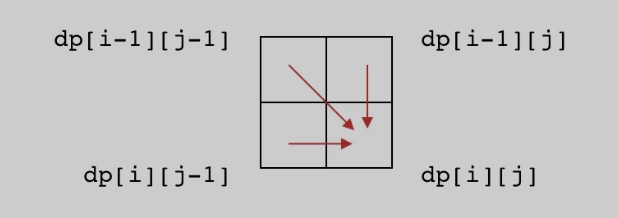# 一些简单动态规划的分析

### 解题思路

• 定义子问题
• 写出子问题的递归关系
• 确定DP数组的计算顺序
• 空间优化(可选)

#### 定义子问题

• 原问题要能够由子问题表示。比如这道题，k=n的时候就是问题的解，不然的话子问题白解了。
• 一个子问题的解要能够由其他子问题的解求出。也就是最优子结构，例如本题的解为$f(k)$可以由$f(k-2)$和$f(k-1)$组成。如果没有这个性质，可能没有办法使用动态规划来解。

#### 写出子问题的递归关系

• 最后一个房子nums[k-1]不偷。那么问题就会变成偷前k-1个房子中偷到最大金额；
• 偷了第k个房子，那么为了满足题目的要求，第k-1个房子就不能再偷了。那么问题就演变成了前k-2个房子偷到最大的金额。

• 当$k=0$时候，即没有房子可以偷，$f(0)=0$；
• 当$k=1$时候，仅有一个房子，直接偷了这个房子，$f(1) = nums$。

### LeetCode 1143

#### 解题步骤

##### 子问题的递推关系

• 如果两个字符相等即s[i-1]==t[j-1]，我们可以用这个字符作为最长公共子序列的最左字符，然后找s的前i-1和t的前j-1个字符的公共子序列；
• 如果两个字符不相等，可以尝试删除s或者t末尾的一个字符，即s的前i-1和t的前i或者s的前i和t的前j-1。再从两种方案中选一个较长的公共子序列。

$$f(i,j)= \begin{cases} f(i-1,j-1) + 1 & if(s[i-1] = t[i-1]) \ max\begin{cases} f(i-1, j)\ f(i, j-1) \end{cases} & other \end{cases}$$

##### 确定DP数组的计算顺序

• DP数组的有效范围是什么？
• 基础解和原问题在DP数组中处于什么位置？
• DP数组的子问题依赖方向是什么？### LeetCode 53

#### 解题步骤

##### 子问题的递推关系

$$f(k)= max \begin{cases} f(k-1) + nums[k-1] \ nums[k-1] \end{cases}$$

$$f(k) = max(f(k-1), 0) +nums[k-1]$$

### LeetCode 718

#### 解题思路

• 子序列 (subsequence) 可以是不连续的。例如 “ACD” 是 “ABCDE” 的子序列；
• 子数组 (subarray)、子串 (substring) 必须是连续的。例如 “BCD” 是 “ABCDE” 的子数组/子串。

##### 子问题的递推关系
• 如果s[i-1] == t[j-1]，那么已经有一个元素是相同的，接下来继续往前看s[0…i-1]和t[0…j-1]的最后几个元素是相同的。也就是$f(i,j)=f(i-1,j-1)+1$。
• 如果s[i-1] != t[j-1]，那么最后一个元素就不相同，前面就不用看了，$f(i,j) = 0$。

$$f(i,j)= \begin{cases} f(i-1,j-1)+1 & if s[i-1] = t[i-1] \ 0 & other \end{cases}$$

### 子问题拆分

https://mp.weixin.qq.com/s/TE-p4WYQBBubhxmT137O9w

https://mp.weixin.qq.com/s/e6L2tvWOS_D0obHR_360Gw